Courses

# JEE(MAIN) Physics Mock Test - 2

## 30 Questions MCQ Test JEE Main Mock Test Series 2020 & Previous Year Papers | JEE(MAIN) Physics Mock Test - 2

Description
This mock test of JEE(MAIN) Physics Mock Test - 2 for JEE helps you for every JEE entrance exam. This contains 30 Multiple Choice Questions for JEE JEE(MAIN) Physics Mock Test - 2 (mcq) to study with solutions a complete question bank. The solved questions answers in this JEE(MAIN) Physics Mock Test - 2 quiz give you a good mix of easy questions and tough questions. JEE students definitely take this JEE(MAIN) Physics Mock Test - 2 exercise for a better result in the exam. You can find other JEE(MAIN) Physics Mock Test - 2 extra questions, long questions & short questions for JEE on EduRev as well by searching above.
QUESTION: 1

### Photons of energy 1eV and 2.5 eV successively illuminate a metal whose work function is 0. 5 eV. The ratio of the maximum speeds of the electrons emitted will be

Solution: Work function=0.5
for 1ev photon
1ev=0.5+kinetic energy
k.E=0.5ev
for 2.5ev photon
2.5ev=0.5ev+k.E
k.E=2ev
ratio of above2 k.E give ratio of square of velocity =1:4
taking square root
finelly the ratio of velocity =1:2ans
QUESTION: 2

Solution:
QUESTION: 3

### The material which practically does not show elastic after effect is

Solution: When an elastic body is stretched and applied deforming force is removed then the body is expected to return to its original configuration instantaneously. This elastic after effect is very short for quartz fiber. But elastic after effect is more for glass.
QUESTION: 4

The average power dissipation in a pure capacitor in AC circuit is

Solution:
Why Power in pure Capacitive Circuit is Zero (0)
Pure inductive circuit, current is lagging by 90 degree from voltage ( in other words, Voltage is leading 90 Degree from current) i.e the pahse difference between current and voltage is 90 degree.
So  If Current and Voltage are 90 Degree Out of Phase, Then The Power (P) will be zero. The reason is that,
We know that Power in AC Circuit
P= V I Cos φ
if angle between current and Voltage are 90 ( φ = 90) Degree. then
Power P = V I Cos ( 90) = 0
[ Note that Cos (90) = 0]So if you put Cos 90 = 0→Then Power will be Zero (In pure Inductive circuit)
QUESTION: 5

A boat crosses a river from port A to port B, which are just on the opposite side. The speed of the water is vW and that of boat is vB relative to water. Assume vB = 2vW. What is the time taken by the boat, if it has to cross the river directly on the AB line?

Solution: Let the velocity of the boat, if it has to cross the river directly on water the line AB be v and the angle between and vB be θ. Then, from the figure sinθ=vwvB Given, vB=2vw ∴ sinθ=vw2vw=12 ⇒ θ=30o Now, the time is taken by the boat to cross the river directly from A to B t=DvA=DvBcosθ =DvBxcos30o or t=2DvB√ 3
QUESTION: 6

The root mean square velocity of the molecules in a sample of helium is 5/7th that of the moelcules in a sample of hydrogen. If the temperature of hydrogen sample is 0oC, then the temperature of the helium sample is about

Solution:
QUESTION: 7

The electric potential at a point (x, y) in the xy-plane is given by: V = -kxy. The electric field intensity at a distance r from the origin varies as

Solution:
QUESTION: 8

A student has measured the length of a wire equal to 0.04580 m. This value of length has the number of significant figures equal to

Solution: There are 4 significant figures. 0’s occurring the to the right of a decimal point and before a non-zero digit is significant and non-zero digits are always considered significant but after the non-zero digit is not considered as significant figures.
QUESTION: 9

The capacity of a parallel plate condenser is 10 μ Fd without dielectric. After that, a dielectric of constant 2 is used to fill half thickness between the plates of another capacitor of same capacitance. The net capacitance after joining both of them in series in μ Fd is

Solution:

Initial c = 10

let capacitance of capictor with dielectric is C1 and without dielectric is ç2

finally they are connected in series

therefore Ceq= C1*C2/(C1+C2)

C1=2*10*2=40

C2=10*2=20

Hence Ceq = 40*20/(20+40)

800/60

=13.33.

QUESTION: 10

If the earth is at one-fourth of its present distance from the sun, then duration of the year will be

Solution: BY Keplers's 3rd law......

Time period T^2=kR^3.

where R is distance of the earth from sun..

so if R'=R/4

T'²=KR'³

T'²=KR³/64

T'²=T²/64

T'=T/8
QUESTION: 11

In the following question, a Statement of Assertion (A) is given followed by a corresponding Reason (R) just below it. Read the Statements carefully and mark the correct answer-
Assertion(A): In a meter bridge, if its wire is replaced by another wire having same length, made of same material but having twice the cross-sectional area, the accuracy decreases.
Reason(R): If its wire is replaced by another wire of same material, having same cross-sectional area but of twice the length, accuracy increases.

Solution:
QUESTION: 12

In a nuclear reactor heavy water is used as a

Solution: Heavy water is used in certain types of nuclear reactors, where it acts as a neutron moderator to slow down neutrons so that they are more likely to react with the fissile uranium-235 than with uranium-238, which captures neutrons without fissioning.
QUESTION: 13

A disc is placed on a surface of pond which has refractive index 5/3. A source of light is placed 4 m below the surface of liquid. The minimum radius of disc needed so that light is not coming out is

Solution: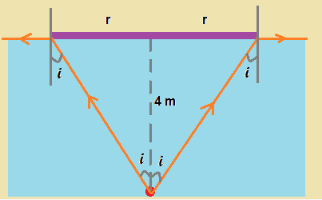We need to block the light rays using the disc till total internal reflection takes place.

As shown in figure, the direction of light ray at the edge of disc is parallel to light surface, when the incident ray makes critical angle
After the edge, light rays will be reflected due to total internal reflection and the whole radiation from light source is blocked

Hence the angle i shown in figure should be critical angle.
For critical angle we have,  sin i = 1/μ = 3/5

hence tan i = 3/4 ....(1)

From figure, we see tan i = r/4  ....(2)

hence from (1) and (2),  the required radius = 3 m
QUESTION: 14

In the following question, a Statement of Assertion (A) is given followed by a corresponding Reason (R) just below it. Read the Statements carefully and mark the correct answer-
Assertion(A): Torque is an axial vector and directed along the axis of rotation.
Reason(R): Torque is equal to vector product of force to the position vector.

Solution:
QUESTION: 15

In order to double the frequency of the fundamental note emitted by a stretched string, the length is reduced to 3/4 th of the original length and the tension is changed. The factor by which the tension is to be changed is

Solution:
QUESTION: 16

Three sound waves of equal amplitudes have frequencies v − 1 , v , v + 1. They superpose to give beats. The number of beats produced per second will be-

Solution:
p1 =po sin 2π(x- 1)t
p2 =po sin 2π(x)t
p3 =po sin 2π(x+ 1)t
p=p1+p3+p2
=posin2π(x-1)t +po sin 2π(x+1)t +po sin 2π(x)t
= 2posin2πxtcos2πt +posin2πxt
= 2posin2πxt[2cos πt +1]
⇒ fbeat = 2
QUESTION: 17

70 calories of heat are required to raise the temperature of 2 moles of an ideal gas at constant pressure from 30oC to 35oC. The amount of heat required to raise the temperature of the same sample of the gas through the same range at constant volume is nearly (Gas constant = 1.99 cal/K-mole)

Solution:
QUESTION: 18

Water is flowing on the blades of a turbine at a rate of 100 kg-s-1 from a certain spring. If the height of the spring be 100 m, then power transferred to the turbine will be

Solution:
Work done = Force X Distance.

From the question :

Force = 100 X 10 = 1000N

Distance = 100m

Work done = 100 X 1000 = 100000Joules

Power = Work done /Time

The time = 1 second.

Power = 100000/1 = 100000 Watts

= 100kW

QUESTION: 19

If the two slits in Young's experiment have width ratio 1:4, the ratio of intensity at maxima and minima in the interference pattern in

Solution: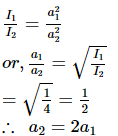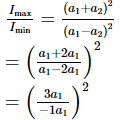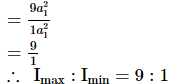QUESTION: 20

Eight negative charges each of value q coulomb are placed at the corners of a cube of side 'a' metre, at the centre of which a positive charge of +2q coulomb is placed.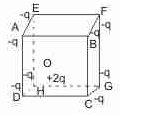Q.
The central charge 2q makes 8 similar pairs with the charges at corners. Their potential energy is :

Solution:
QUESTION: 21

Eight negative charges each of value q coulomb are placed at the corners of a cube of side 'a' metre, at the centre of which a positive charge of +2q coulomb is placed.Q.
Each charge is at a distance 'a' metre from three neighbouring charges, such as A is at a distance 'a' metre from E, B and D and so on. However, each pair is repeated when we consider all pairs such as AE and EA. The potential energy of these pairs is :

Solution:
QUESTION: 22

Eight negative charges each of value q coulomb are placed at the corners of a cube of side 'a' metre, at the centre of which a positive charge of +2q coulomb is placed.Q.
Each charge is at a specific distance from the diagonally opposite charges, such as A is at a specific distance from C, F and H and similarly H is at a specific distance from A, C and F and so on. Each pair is repeated here also. The potential energy of these pairs is :

Solution:
QUESTION: 23

In parallel grouping of cells equivalent emf is given by: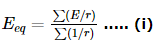If polarity of any of the cells is reversed, then in the mumerator of Eq.(i) negative sign comes at that place.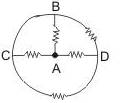Q.
Five identical cells are connected in parallel. Now, polarity of one of the cells is reversed. Percentage change in equivalent emf will be:

Solution:
QUESTION: 24

In parallel grouping of cells equivalent emf is given by:If polarity of any of the cells is reversed, then in the mumerator of Eq.(i) negative sign comes at that place.Q.
In the circuit shown in figure, each resistance is R. Net resistance between points A and B is:

Solution:
QUESTION: 25

In an optics experiment, with the position of the object fixed, a student varies the position of convex lens and for each position, the screen is adjusted to get a clear image of the fine object. A graph between the object distance u and the image distance v, from the lens, is plotted using the same scale for the two axes. A straight line passing through the origin and making an angle of 450 with the x - axis meets the experimental curve at P. The coordinates of P will be:

Solution:
QUESTION: 26

A sphere of mass m moving with constant velocity u, collides with another stationary sphere of same mass. If e is the coefficient of restitution, the ratio of the final velocities of the first and second spheres is

Solution:
QUESTION: 27

A mass of 0.5 kg moving with a speed of 1.5 m/s on a horizontal smooth surface, collides with a nearly weightless spring of force constant k = 50 N/m. The maximum compression of the spring would be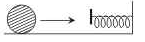Solution: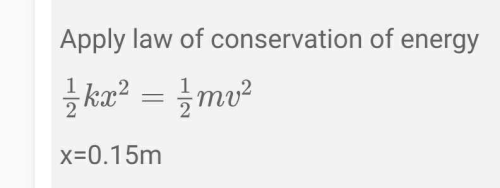QUESTION: 28

A uniform disc of radius R lies in the x - y plane, with its centre at origin, its moment of inertia about z-axis is equal to it moment of inertia about line y = x + c. The value of c will be

Solution:
QUESTION: 29

The figure shows certain wire segments joined together to form a coplanar loop. The loop is placed in a perpendicular magnetic field in the direction going into the plane of the figure. The magnitude of the field increases with time. I1  and I2 are the currents in the segments ab and cd. Then,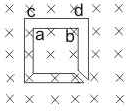Solution: According to Lenz’s law, current will flow in anticlockwise direction as magnetic field is increasing into the plane of paper.
QUESTION: 30

One end of a string of length L is tied to the celling of a lift accelerating upwards with an acceleration 3g. The other end of the string is free. The linear mass density of the string varies linearly from 0 to λ - from bottom to top. Mark out the correct statement for a wave pulse travelling on the string.

Solution: